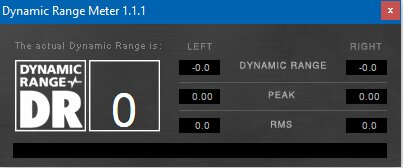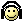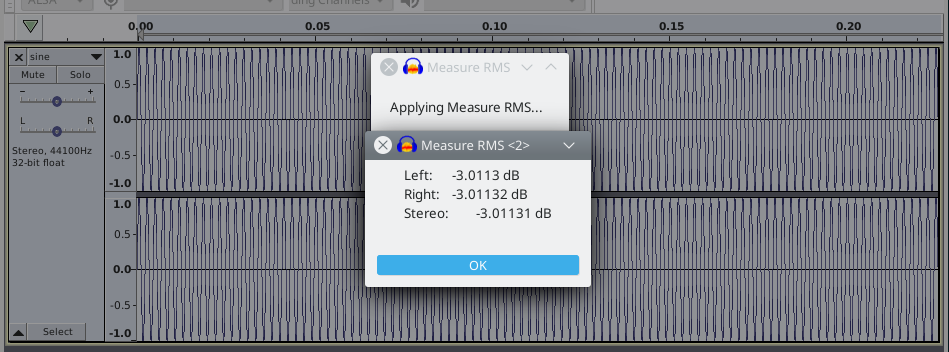Foobar's dynamic range meter
post-15843556

#### VNandor

I've just checked the "dynamic range" of a 1 minute long 0dBFS 1kHz sine wave. I was expecting the meter to report a -3dB RMS and probably 0dB dynamic range. Instead, I got a 0dB RMS and 0 dynamic range. Am I wrong to expect a -3dB RMS reading from this "test"? If so, why is that? Does anyone know what the program actually reports as RMS?

post-15843614

#### castleofargh

##### Sound Science Forum Moderator
Not sure I understand this right. Your signal remains at the same level(0dBFS but that doesn't matter), so if you look at a meter, you'll constantly read the same RMS level. But that's not what you're reading here. You're reading a measure of the dynamic range! which in this case seems to be some measure of variation in the RMS levels. That level does not change over time with that particular test signal, so the dynamic range is zero.

does this clear the confusion?

post-15843622

#### VNandorIm expecting the dynamic range to be 0, the peak to be 0 and the RMS of the signal to be at -3dB because that is the RMS of a sine wave.

post-15843760

Because the "RMS" calculated in the algorithm is not a real RMS but:
the square root of the double sum over all input samples squared, divided by the block size in samples
(emphasis mine)

Why? My guess:
• they wanted DR value of pure sine to be 0,
• the DR value in the algorithm is calculated as a difference between the peak and that pseudo RMS,
• so if they used an actual RMS the difference wouldn't be 0.

•castleofargh and VNandor
post-15843808

A sine wave has peaks and values. That's why the RMS value has to square it and then divide. What you want is the average (RMS = Root Mean Squared) not the peak.post-15843852

#### VNandor

Because the "RMS" calculated in the algorithm is not a real RMS but:

(emphasis mine)

Why? My guess:
• they wanted DR value of pure sine to be 0,
• the DR value in the algorithm is calculated as a difference between the peak and that pseudo RMS,
• so if they used an actual RMS the difference wouldn't be 0.
Ah, thank you. So if I understand the calculations correctly, subtracting 3dB from what the program reports should give the actual RMS most of the time, is that right? Because the multiplication of sqrt(2) of the samples has the same effect as adding ~3dB to the end result I think.

The only time this wouldn't work is if the signal's actual RMS is already more than -3dB RMS. I mean a square wave with an amplitude of 0.8 has a ~ -2dB RMS while an amplitude of 0.9 would give ~ -0.9dB RMS. Both of these signals are reported as 0dB RMS by foobar.

The reason I came across this is because I used this RMS value to volume match while testing and with some of the music I could still hear some volume difference. At least now I know it doesn't work properly with some of the (extremely loud) music I have.

post-15843863

#### VNandor

A sine wave has peaks and values. That's why the RMS value has to square it and then divide. What you want is the average (RMS = Root Mean Squared) not the peak.Yes, I'm aware of the definition of RMS. The reason foobar confused me is because it doesn't use the standard definition of RMS.

post-15846454

Ah, thank you. So if I understand the calculations correctly, subtracting 3dB from what the program reports should give the actual RMS most of the time, is that right? Because the multiplication of sqrt(2) of the samples has the same effect as adding ~3dB to the end result I think.

The only time this wouldn't work is if the signal's actual RMS is already more than -3dB RMS. I mean a square wave with an amplitude of 0.8 has a ~ -2dB RMS while an amplitude of 0.9 would give ~ -0.9dB RMS. Both of these signals are reported as 0dB RMS by foobar.
Yes, I think so.

I wasn't sure if that displayed value is for the whole track or for the 20% of the loudest 3-second blocks (that's what is actually used to calculate the DR value), but it looks like it is for the whole track.

The reason I came across this is because I used this RMS value to volume match while testing and with some of the music I could still hear some volume difference. At least now I know it doesn't work properly with some of the (extremely loud) music I have.
I use either SoX:
Code:
``````]\$ sox sine.wav -n stats
Overall     Left      Right
DC offset   0.000000  0.000000 -0.000000
Min level  -0.999908 -0.999908 -0.999908
Max level   0.999908  0.999908  0.999908
Pk lev dB      -0.00     -0.00     -0.00
RMS lev dB     -3.01     -3.01     -3.01
RMS Pk dB      -3.00     -3.00     -3.00
RMS Tr dB      -3.06     -3.06     -3.06
Crest factor       -      1.41      1.41
Flat factor     0.00      0.00      0.00
Pk count        11.5        10        13
Bit-depth      16/16     16/16     16/16
Num samples    22.0k
Length s       0.500
Scale max   1.000000
Window s       0.050``````
or Audacity (its Measure RMS tool has to be enabled manually):•VNandor
post-15853812

#### playmusic

Because the "RMS" calculated in the algorithm is not a real RMS
Im expecting [...] the RMS of the signal to be at -3dB because that is the RMS of a sine wave.
Yes, I'm aware of the definition of RMS. The reason foobar confused me is because it doesn't use the standard definition of RMS.
It might surprise you, but the above discussed rms calculation in foobar (precisely the Dynamic Range component for foobar) for a sine wave is correct if you look at it in a quite plausible context.

The "dB" is always about a relative measure.
The full scale signal level is defined in the standard AES-17 as relative to a full-scale sine wave.
The standard even mentions explicitly that a square wave can read as much as +3.01 dB FS.
So, in the context of AES standards, the algorithm in foobar is correct about the rms of a sine wave.

At the same time, there seems to be for signals with a positive dB FS rms a bug in the software implementation of foobar's algorithm.
To me this bug is not relevant for music listening because a song with a positive dB FS rms (according to AES definition) is rather unappealing, I think, since it is something between a FS sine [edited: "and"] a maximum amplitude square wave.

But music tastes vary as evidenced in this post about music with (it seems) a positive dB FS rms where foobar goes wrong:
The reason I came across this is because I used this RMS value to volume match while testing and with some of the music I could still hear some volume difference. At least now I know it doesn't work properly with some of the (extremely loud) music I have.

Last edited:
•